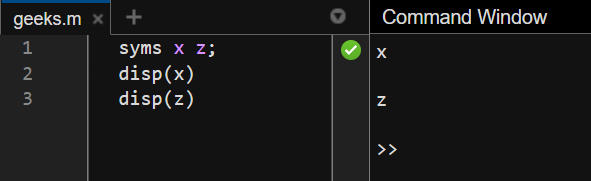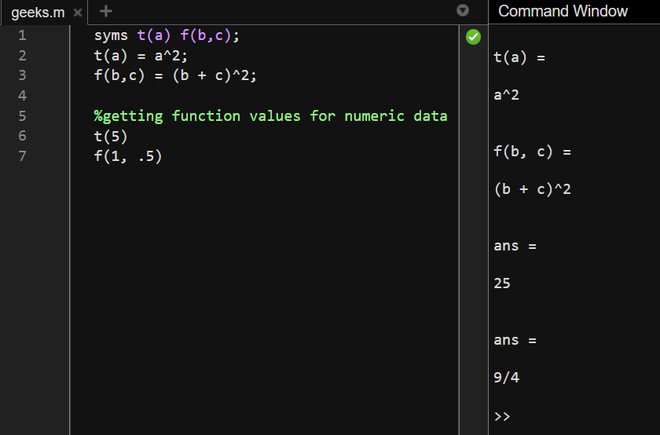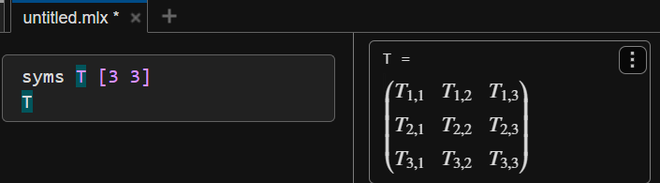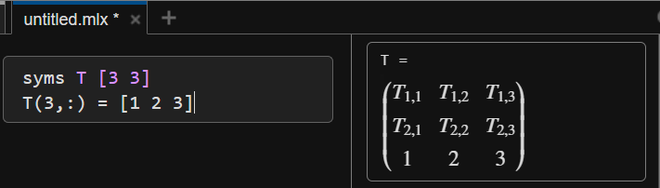GeeksforGeeks App
Open AppBrowser
Continue

## Related Articles

Symbolic variables in MATLAB are variables that store a literal, such as x, \$, as a symbol. This means that whatever a symbolic variable stores are not a value the computer can compute until it is assigned a numeric value. It is just a symbolic representation. To understand the same, see the following case.
If a user needs to display an equation like follows:

x = x + 1

This can be done with the usage of symbolic variables. One would make a symbolic variable, say x and assign them values x + 1. Then, typing the following will display the above equation.

x = x + 1

Now, we shall see how to create variables, functions, and matrix symbolic variables with an example of each.

## Scalar Symbolic Variable:

To create a symbolic scalar variable, the syms keyword can be used.

Syntax:

syms <variable list>

Example 1:

## Matlab

 `% MATLAB Code``syms x z;``disp(x)``disp(z)`

Every variable created will have a symbolic value, the same as its name.

Output:## Symbolic Functions:

Symbolic functions work upon symbolic variables and can be used for displaying and computing numeric calculations.

Syntax:

syms <function name>(parameters)

This will create a symbolic function. See the following example for a better understanding.

In this example, we create two symbolic functions. The first one computes the square of a variable and the second one computes the whole square of the sum of two variables.

Example 2:

## Matlab

 `% MATLAB Code``syms t(a) f(b,c);``t(a) = a^2;``f(b,c) = (b + c)^2;`` ` `% Getting function values for numeric data``t(5)``f(1, .5)`

The output of the above code first displays the symbolic representation of the two functions and then computes the values of these functions for a given numeric data.

Output:## Symbolic Matrix:

A symbolic matrix will have representation like it is done in abstract algebra. To create such a matrix, the syms keyword takes the following form:

syms <matrix name> [size vector]

See the following example to understand the same. We will create a matrix named T of size 3×3.

Example 3:

## Matlab

 `% MATLAB Code``syms T [3 3]``T`

Output:As can be seen, the elements in matrix T are represented in the following form.

Trow number, column number

We can then change any element using the array indexing methods.

Example 4:

## Matlab

 `% MATLAB Code``syms T [3 3]``T(3,:) = [1 2 3]`

In this example, we change the third row from symbolic to numeric data. The output of above code is:

Output:## Conclusion

In this article, we discussed how to create symbolic scalar variables, functions, and matrix variables in MATLAB with the help of syms function.

My Personal Notes arrow_drop_up